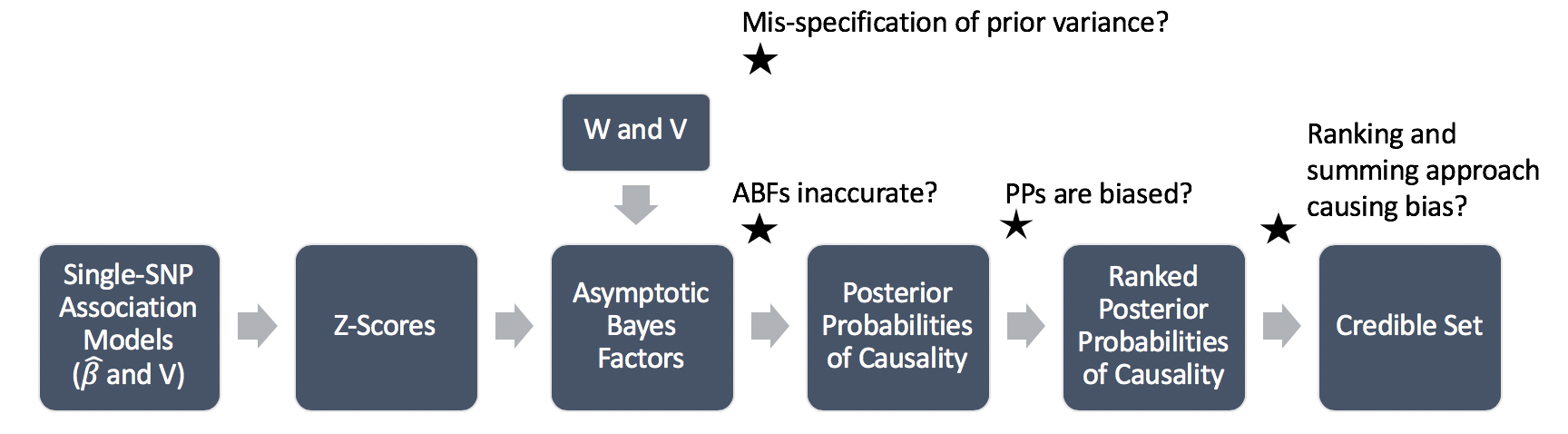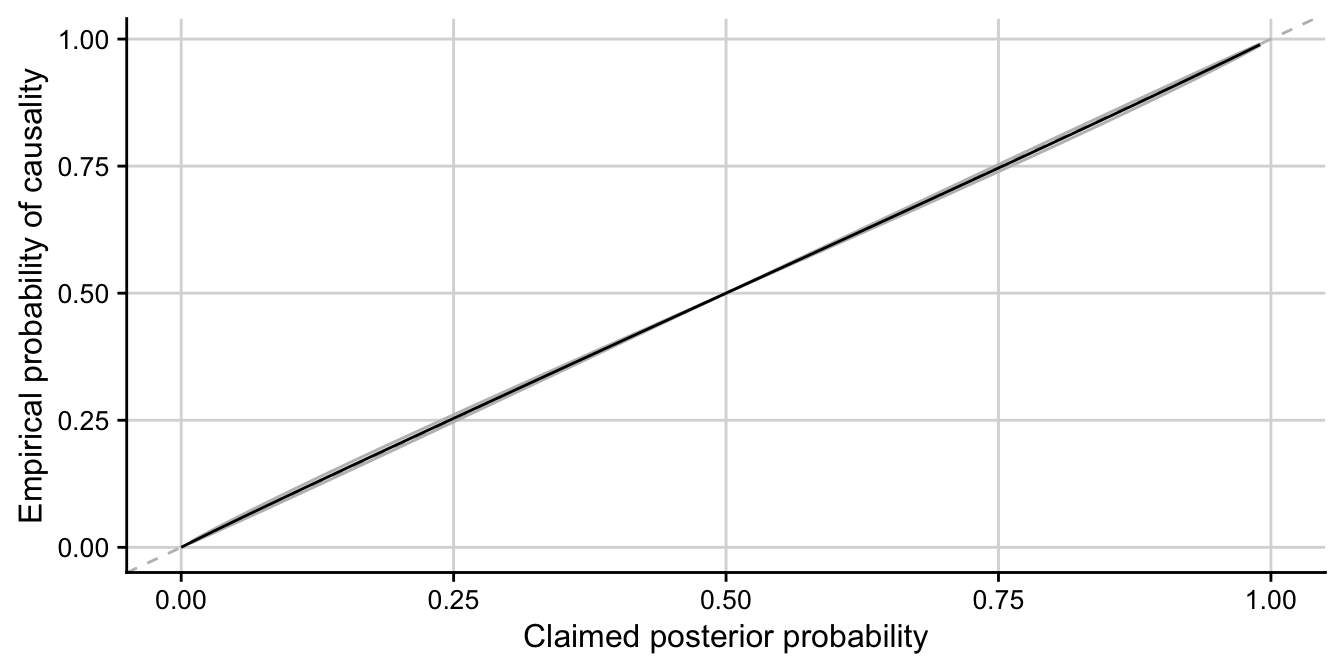### Motivation

We have found there to be systematic bias in the coverage estimates of the credible sets comprising of putative causal variants in single causal variant Bayesian genetic fine-mapping. The procedure of forming these credible sets is outlined below, with asterisks marking possible causes/contributors to this bias.We think that it is unlikely that the prior variance has been mis-specified or that the ABFs are inaccurate. We therefore start with the third asterisk and investigate whether the PPs themselves are biased. To do this, we simulate some data and plot the estimated probability of causality against the claimed posterior probability (PP). The following plot is the prediction from a $$logit(y)\sim logit(x)$$ model fitted to 10000 simulations where $$y$$ is a binary indicator of SNP causality and $$x$$ is the claimed posterior probability. We find that the PPs are well empirically calibrated themselves, revealing that it is likely the method of forming the credible sets (sort and sum) which is causing the bias.We hypothesize that the problem could be related to the flat prior that is routinely used in the method, whereby the prior probability of causality is flat across all SNPs. Whilst this is accurate to calculate the per-SNP posterior probabilities of causality, it seems unrealistic to maintain this “flat belief” once the SNPs have been sorted into descending order of posterior probability. This motivates the idea of considering a “credible set prior” that depends on the ordering of the SNPs.

### The Dirichlet distribution

The flat prior probability of causality across all SNPs is the expectation of a Dirichlet distribution with parameter $$\mathbf{\alpha}=\mathbf{1}$$. We consider taking samples from this.

Input to Dirichlet: Any vector of length $$k$$,

Output of Dirichlet: A vector of length $$k$$ that defines a probability distribution (probabilities that sum to 1).

##' Function to generate random deviates from the Dirichlet distribution.
##'
##' @title
##' @param n number of random vectors to generate
##' @param a vector containing concentration parameters
##' @return
rdirichlet<-function(n, a){
l <- length(a);
x <- matrix(rgamma(l*n,a),ncol=l,byrow=TRUE); # generate l*n rgamma random quantities
sm <- x %*% rep(1, l); # calculate row sums
x/as.vector(sm); # normalise to sum to one
}

nsnps <- 100
x <- rdirichlet(10000, rep(1, nsnps))
plot(colMeans(x), ylim = c(0, 0.02)) # will tend to 1/nsnps
abline(h = 0.01, col = 2)